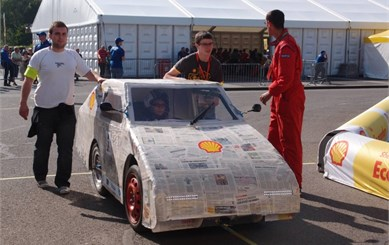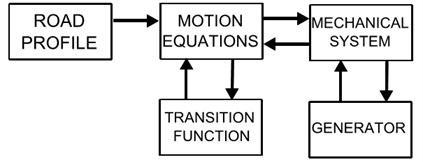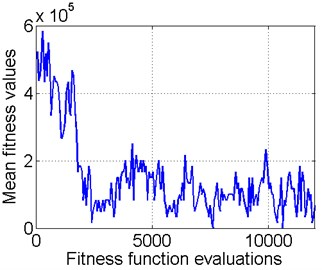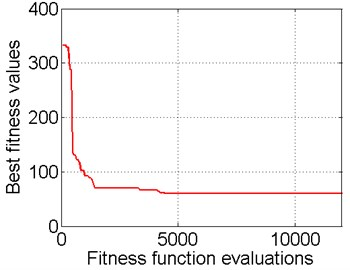Published: 10 October 2014

# The optimization of the parameters of the energy recovery device from vibration of a vehicle suspension

Wojciech Skarka1
Mateusz Tyczka2
Piotr Przystałka3
1, 2, 3Institute of Fundamentals of Machinery Design, Faculty of Mechanical Engineering, Silesian University of Technology, Gliwice, Poland
Corresponding Author:
Wojciech Skarka
Views 77

#### Abstract

The paper presents the numerical optimization model of the energy recovery system from a suspension of a racing vehicle. The system is based on the recovery of energy lost in the classical solutions in the viscous damper. The suspension system should provide adequate comfort to a driver, while allowing recovery of the maximum amount of energy. The goal of the research is to draw general conclusions about the optimal parameters for similar systems. The parameters selection for the energy recovery system is a difficult task. For this reason, it has been decided to build a simulation model that allows the selection of the optimal parameters of the system. The fitness function of the model is the amount of energy recovered from the system. The amount of recovered energy should be as high as possible, while ensuring a good comfort for a driver, which is necessary, to recognize the result as valid. During the optimization each set of parameters has been simulated for different road profiles with different road surface quality. The model has been prepared for the racing car, but its universality allows for the selection of optimal parameters of energy recovery system for other vehicles.

## 1. Introduction

Decreasing amount of fossil fuels makes it necessary to constantly increase the effectiveness of their use. This also applies to vehicles. One of the possibilities to improve the efficiency of cars is recovering energy from the vehicle’s suspension. The idea of recovering energy from the suspension is not brand new. Energy recovery from the suspension, means replacing the classical shock absorbes with the element, that allows for the recovery of dissipated energy. There are many possible solutions. The most popular are the solutions using electrohydraulics energy recovery [1, 2]. Other solution is to use a linear electric motor . A large group of solutions is based on electromechanical system and a DC motor as a power generator [4, 5]. As in the case of , the authors have decided to use a rack gear and the DC motor as a generator for energy recovery. In addition, it has been decided to introduce a mechanism guaranteeing a constant direction of rotation of the motor shaft and the additional multiplication speed of the motor shaft. Determination of optimal parameters for energy recovering system is difficult. In this paper the focus is to define the general conclusions concerning the parameters of this type of energy recovery unit. For this purpose, a mathematical model of system has been determined and then implemented in MATLAB SIMULINK® calculation software. For calculation has been used a numerical model of a racing car [6, 7] (Fig. 1), prepared to take part in competitions Shell Eco-Marathon . The research has a preliminary nature. It was decided to use the popular vehicle quarter car model [9, 10]. Due to the large number of possible combinations the genetic algorithm, has been used, which has already been applied in similar studies [10, 11], to calculate the optimal parameters. In addition to energy recovery, which is the main criterion of the optimization, also verified the comfort of the driver has been included, which is a secondary criterion due to the nature of the example. Range of parameters has been chosen based on previous studies on the use of energy recovery from a suspension and the vehicle energy model of a similar vehicle [12-15].

Fig. 1Racing vehicle prepared for Shell Eco-marathonFig. 2Structure of the simulation model## 2. Simulation model

In order to determine the optimal parameters, it was necessary to create a mathematical model of the vehicle. Due to the nature of the initial research, it has been decided only to verify driver comfort. Adequate to do this is the quarter car model [9, 10]. The model consists of unsprung mass representing the weight of the wheel and sprung mass which is one quarter of the weight of the vehicle body and the driver. The tire of the vehicle has been described by parallel-connected spring and damping elements with fixed parameters. The suspension has been defined by the spring element of constant parameters. In the equations the damping force has been introduced arising from the generation of energy through energy recovery system. Additionally, the impact of the suspension arm used in the vehicle has been taken into account. In the case of the car for which studies were performed the simple suspension arm was used, which allowed the introduction of simple mathematical relations. Energy recovery system consists of a mechanical system and a DC motor working as a generator. The mechanical system has the task of maintaining a constant direction and speed multiplication of the motor shaft. The mathematical equations describing the model are shown below:

1
${m}_{1}{\stackrel{¨}{x}}_{1}+F+{c}_{1}\left({x}_{1}-{x}_{2}^{\text{'}}\right)=0,$
2
${m}_{2}{\stackrel{¨}{x}}_{2}-F-{c}_{1}\left({x}_{1}-{x}_{2}^{\text{'}}\right)+{b}_{2}\left({\stackrel{˙}{x}}_{2}^{\text{'}}-\stackrel{˙}{w}\right)+{c}_{2}\left({x}_{2}^{\text{'}}-w\right)=0,$
3
$F=i{K}_{2}r\frac{1}{n},$
4
$i{R}_{z}={K}_{1}\left|{x}_{1}-{x}_{2}^{\text{'}}\right|r-iR-L\frac{di}{dt},$

where: subscript is 1 for sprung mass and 2 is for unsprung mass, $m$ is for mass, $x$ is for displacement, $w$ is for road profile, $c$ is for spring rate, $b$ is for damping factor, $F$ is damping force arising from the energy generator, apostrophe like ${x}_{2}^{\text{'}}$ is function of displacement arising by suspension arm, ${R}_{z}$ is for external resistance, $r$ is total ratio of the mechanical system, $R$, ${K}_{1}$, ${K}_{2}$, $L$ are parameters of DC engine used for generating energy, $n$ is efficiency of mechanical system.

In order to determine the optimal parameters, the system has been modeled in the MATLAB SIMULINK® software. The input to the model is the road profile. The road profile has been generated at random, based on the PSD [16-18] in accordance with the standard . 10 cases have been verified. For each, three types of surfaces of different quality have been tested. The model has been divided into blocks. The mechanical system and generator are blocks responsible for energy recovery system. The transition function is the block responsible for calculating displacement which takes into account the suspension arm. A schematic structure of the model is shown in Fig. 2.

The main purpose of the optimization process is to adjust parameters such as ${c}_{1}$, ${z}_{1}$, ${z}_{2}$, ${z}_{3}$, $p$ and to find suitable type of a motor in order to minimize the cost of function $U$, which can be formulated taking into account the following two criteria. The first criterion is to recover maximal quantity of the energy, whereas the second one is to minimize the influence of car vibrations. The authors propose to use the global criterion method  in which two objectives are combined. One of the most general indirect utility functions at this matter can be expressed in its simplest form as the weighted exponential product:

5
$U\left(\mathbf{q}\right)={\frac{1}{{\left(\sum _{i=1}^{r}\sum _{j=1}^{N}{P}_{j}\right)}^{{w}_{1}}}\left[{\sum }_{i=1}^{r}\left(1E6\bullet H\left({A}_{i}-ϵ\right)+{A}_{i}\right)\right]}^{{w}_{2}}+\delta ,$

where $\mathbf{q}=\left[{c}_{1}{z}_{1}{z}_{2}{z}_{3}pMotorID\right]$, ${w}_{1}$ and ${w}_{2}$ denote weights indicating the relative significance of the criterion functions, $H$ is the Heaviside step function, $ϵ$ is a threshold corresponding to the EU rules, $\delta$ is a penalty component (it is equal to 1E6 if ${z}_{2}$ and ${z}_{3}$ are simultaneously even or odd), ${P}_{j}$ is the estimation of the recover power, $r$ is the number of the road surface types, $N$ is the number of samples corresponding to the length of the simulation. Parameters $p$, ${z}_{1}$, ${z}_{2}$, ${z}_{3}$ describe the mechanical system of energy recovery system. The influence of the vibration of the vehicle on a driver is evaluated using the estimation of the root mean square of the acceleration signal of the car suspension [20, 21]:

6
${A}_{i}=\frac{1}{N}\sqrt{{\sum }_{j=1}^{N}{a}_{j}^{2}}.$

A large number of algorithms can be used for solving the problem stated above. However, standard optimization methods e.g. gradient-based approaches cannot be adopted in this context, mainly due to the form of the cost function (Eq. (1)) and because there are continuous and discrete independent variables in the vector $\mathbf{q}$. For these reasons, the authors decided to apply evolutionary optimization algorithms which are based on the natural selection process that mimics biological evolution. Well-known genetic operators for single-objective optimization are used to guarantee the convergence of a solution .

## 4. Result

In the present study, the optimization process was carried out using MATLAB® with Genetic Algorithm and Direct Search toolbox. The behavioural parameters of the evolutionary algorithm were chosen according to the guidelines suggested in the literature . The fitness function was based on the meta-criterion (Eq. (5)), wherein the weights were the same for both criteria (${w}_{1}=$0.5, ${w}_{2}=$0.5) and the parameter $ϵ=$3.25 (taking into account restrictions in the UE law) . Due to the preliminary results of the simulation it was decided that the upper and lower limits of the variables ${c}_{1}$, ${z}_{1}$ etc. were chosen taking into account typical and normalized elements and according to parameters of the race car. It was determined that individuals in the population would be composed of genes representing real numeric and integer values. Genes in the chromosome corresponds to the values of the elements of the vector $\mathbf{q}$. The feasible population method was adapted to create a random well-dispersed initial population that satisfies all bounds. The ranking method was used to scale the fitness function and the roulette method was employed to choose parents creating new individuals for the next generation. Reproduction was carried out applying the elite count method (the number of individuals equal to 2) and crossover as well as mutation operators. In the paper, the heuristic crossover was realized with a probability of 0.8. The remaining individuals were processed using an adaptive feasible mutation function. The maximum number of generations was chosen as the criterion for stopping the algorithm. It was experimentally established that, the population size would equal to 60 and a maximum number of generations would equal to 200. The optimization procedure was realized ten times to obtain averaged results which are presented in Table 1. The best trial was achieved in the case of ProfileID $=$ 08. Fig. 2 presents the convergence of the optimization algorithm for this case.

Table 1Results of the optimization process for different variants of the surface of the road

 Profile ID ${c}_{1}$ ${z}_{1}$ ${z}_{2}$ ${z}_{3}$ $p$ Motor ID $U$ 00 9000 17 63 38 150 28 (${K}_{1}=$56, ${K}_{2}=$170, $R=$ 10, $L=$2.62, $J=$ 122) 202 01 19400 29 70 25 150 35 (${K}_{1}=$39.5, ${K}_{2}=$242, $R=$ 3.9, $L=$2.83, $J=$ 560) 98 02 11500 24 70 25 100 35 (${K}_{1}=$39.5, ${K}_{2}=$242, $R=$ 3.9, $L=$2.8, $J=$ 560) 91 03 18500 17 48 17 150 5 (${K}_{1}=$56, ${K}_{2}=$170, $R=$ 9.97, $L=$2.62, $J=$ 120) 90 04 8900 17 35 18 150 28 (${K}_{1}=$56.2, ${K}_{2}=$170, $R=$ 10.2, $L=$2.62, $J=$ 122) 167 05 8800 17 62 17 150 29 (${K}_{1}=$44.7, ${K}_{2}=$214, $R=$ 16, $L=$4.14, $J=$ 124) 80 06 17600 17 68 25 150 28 (${K}_{1}=$56.2, ${K}_{2}=$170, $R=$ 10.2, $L=$2.620, $J=$ 122) 89 07 15000 22 70 17 150 27 (${K}_{1}=$69.7, ${K}_{2}=$137, $R=$ 6.61, $L=$1.7, $J=$ 123) 82 08 11700 17 70 23 150 35 (${K}_{1}=$39.5, ${K}_{2}=$242, $R=$ 3.9, $L=$2.83, $J=$ 560) 60 09 13000 17 69 28 150 28 (${K}_{1}=$56, ${K}_{2}=$170, $R=$ 10.2, $L=$2.62, $J=$ 122) 165

Fig. 3Mean and best fitness function values vs. the number of function evaluationsa)b)

## 5. Conclusions

The best result of fitness function has been achieved for the case of 8. Because the road profiles were generated at random, while retaining a similar road standard, it cannot be concluded that this is the best set of parameters for each surface. However, based on other results, it is possible to draw some general conclusions. The first of the conclusions is the striving for high gears at speed multiplication on the shaft of the generator. This is proved by the results of gear ratios ($p$ parameter). Most of them is 150 which is maximum possible value. On the basis of the parameters ${z}_{1}$, ${z}_{2}$, ${z}_{3}$ (number of gear teeth of a mechanical system) similar conclusions can be drawn. In 9 out of 10 cases, as the optimal engine, a powerful engine has been calculated (150 to 200 W). This indicates that the generator should use a high-power engine. The damping force values obtained from energy recovery system proved to be low. This demonstrates the need for duplication of energy recovery system or using an additional damping system, if used in the vehicle. The research had preliminary nature. Before using the system in the vehicle, further studies are necessary and verification of numerical results for real energy recovery system. The current results are encouraging for further work.